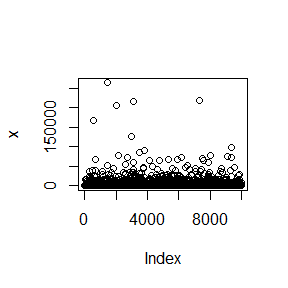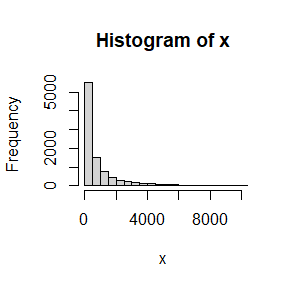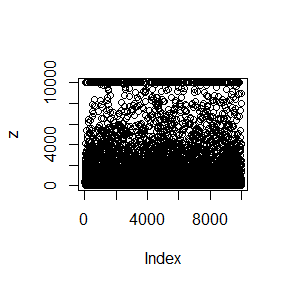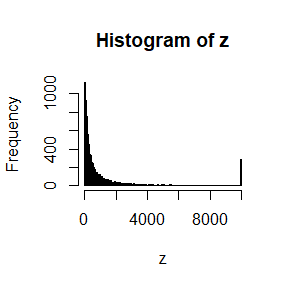# Capped mean, Exposure and Increased Limit Factor curves

## 1.Introduction

This vignette will cover capped mean functions, exposure and increased limit factor curves of the NetSimR package. It will overview the theory behind these functions, propose posible uses and illustrate examples. The distributions covered for claim severities are LogNormal, Gamma, Pareto, Sliced LogNormal-Pareto, Sliced Gamma-Pareto. An application of the capped mean is suggested in the article “Taken to Excess” accessible here.

## 2.Backround theory

Let claim severity $$x$$ originate from a probability density function $$f(x)$$ and a cumulative density function $$F(x)$$. Let claims be capped at amount $$c$$. The capped claim severity is defined as $$y=Min(x,c)$$.

### 2.1 Capped mean

The expected mean can be estimated by: $E(x)=\int_0^{\infty}x*f(x)~dx$ The expected capped claim can be obtained by: $E(y)=\int_0^{\infty}y*f(x)~dx$ The above simplifies to: $E(y)=c-\int_0^{c}F(x)~dx$ This equation will need to be solved for every distribution.

### 2.2 Exposure curve

This function provides the percentage of total claims cost that lies below a certain claims level. Exposure curve at a claims cap is calculated by: $EC(c)=\frac{E(y)}{E(x)}$

### 2.3 Increased limit factor curve

This function provides the ratio of expected capped claims to a higher cap $$c_{h}$$ to the expected claims of a lower cap $$c_{l}$$. Let the claim capped at $$c_{h}$$ be $$y_{h}$$ and the claim capped at $$c_{h}$$ be $$y_{h}$$. Increased limit factor curve at a claims caps $$c_{h}$$ and $$c_{l}$$ is calculated by: $ILF(c_{h},c_{l})=\frac{E(y_{h})}{E(y_{l})}$

## 3. Functions usage

In this section we will suggest possible uses of the specified functions.

### 3.1 Capped mean

• Restricting expected claims from being modelled beyond the property’s value, in property lines.
• Modelling attritional losses with a censored or truncated distribution, in order to improve our estimate. Large claims will be modelled separately. This can be extended to Generalised Linear Models.
• Estimating the cost of deductibles.
• Pricing reinsurance excess of loss layers.

### 3.2 Exposure curve

For property lines/sections:

• Pricing/allocating premium of exess of loss layers.

Note: for property lines, instead of severity, we may opt to model severity as a percentage of sum insured. In GLMs this is equivalent to having the sum insured as the exposure for the severity distribution.

### 3.3 Increased limit factor curve

For casualty lines/sections:

• Pricing/allocating premium of exess of loss layers.

## 4. Examples

### 4.1 Capped mean

The below code ilustrates an alternative and more accurate approach to applying capping in regressions, for modelling attritional claims part. The code steps are:

• Simulate 10,000 claims
• Cap the claims
• Fit a lognormal regression to the capped claims
• Fit a censored regression to the capped claims
• Compare:
• the expected mean from the normal regression
• the expected capped mean from the censored regression
• the mean of the capped claims data

The example suggests that the proposed method outperforms the market approach on LogNormal distribution. The one-way benefit will not be significant on a Gamma distribution, but when we move to a GLM and the model relativities are larger, the capped mean benefit may also be significant.

#required packages
library("NetSimR")
library("crch")

#Set parameters
n<-10000
mu<-6
sigma<-1.7
Cap <- 10000

#Set seed to keep simulations constant
set.seed(10)

#Simulate data
x<-round(rlnorm(n,mu,sigma),0)
head(x)
##  416 295  39 146 666 783
summary(x)
##    Min. 1st Qu.  Median    Mean 3rd Qu.    Max.
##       1     128     400    1769    1279  263389
plot(x)hist(x, breaks = 400, xlim = c(0,10000))#cap data
z<-ifelse(x>Cap,Cap,x)
head(z)
##  416 295  39 146 666 783
summary(z)
##    Min. 1st Qu.  Median    Mean 3rd Qu.    Max.
##       1     128     400    1282    1279   10000
plot(z)hist(z, breaks = 200)#fit linear regression
lmLinear<-lm(log(z)~1)
summary(lmLinear)
##
## Call:
## lm(formula = log(z) ~ 1)
##
## Residuals:
##     Min      1Q  Median      3Q     Max
## -5.9815 -1.1295  0.0099  1.1723  3.2288
##
## Coefficients:
##             Estimate Std. Error t value Pr(>|t|)
## (Intercept)  5.98153    0.01662   359.8   <2e-16 ***
## ---
## Signif. codes:  0 '***' 0.001 '**' 0.01 '*' 0.05 '.' 0.1 ' ' 1
##
## Residual standard error: 1.662 on 9999 degrees of freedom
#fit right censored regression
lmCensored<-crch(log(z)~1, right = log(Cap), link.scale="identity", dist = "gaussian")
summary(lmCensored)
##
## Call:
## crch(formula = log(z) ~ 1, link.scale = "identity", dist = "gaussian",
##     right = log(Cap))
##
## Standardized residuals:
##     Min      1Q  Median      3Q     Max
## -3.5188 -0.6735 -0.0053  0.6763  1.8823
##
## Coefficients (location model):
##             Estimate Std. Error z value Pr(>|z|)
## (Intercept)  6.00054    0.01708   351.2   <2e-16 ***
##
## Coefficients (scale model with identity link):
##             Estimate Std. Error z value Pr(>|z|)
## (Intercept)  1.70526    0.01234   138.2   <2e-16 ***
## ---
## Signif. codes:  0 '***' 0.001 '**' 0.01 '*' 0.05 '.' 0.1 ' ' 1
##
## Distribution: gaussian
## Log-likelihood: -1.936e+04 on 2 Df
## Number of iterations in BFGS optimization: 6
#Compare regression outputs
cbind(coefficients(lmLinear),summary(lmLinear)$sigma) ## [,1] [,2] ## (Intercept) 5.981527 1.662243 coefficients(lmCensored) ## (Intercept) (scale)_(Intercept) ## 6.000537 1.705257 #Compare regressions' attritional capped cost to empirical round(cbind( Empirical=mean(z) ,LinearModel=exp(coefficients(lmLinear)+0.5*summary(lmLinear)$sigma*summary(lmLinear)\$sigma)
,CensoredModel=LNormCappedMean(Cap,coefficients(lmCensored),coefficients(lmCensored))
),0)
##             Empirical LinearModel CensoredModel
## (Intercept)      1282        1577          1284

Modelling excess levels would be similar to the above approach. In that case the expected mean above the excess levels would be the mean less the expected mean below the excess level. This is elaborated in the article cited in the introduction section.

### 4.2 Exposure curve

For a LogNormal severity, generating the exposure curve value at a particular point is obtained by running the below code:

ExposureCurveLNorm(1000,5,1.6)
##  0.5601327

This would be interpreted as: 56% of the expected cost is up to 1,000 currency units. Other distributions follow the same logic. The challenge for this exercise would be fitting the correct severity distribution, rather than calculating the Exposure curve’s value.

### 4.3 Increased limit factor curve

For a LogNormal severity, generating the increased limit factor value between two particular points is obtained by running the below code:

ILFLNorm(1000,1500,5,1.6)
##  1.155086

This would be interpreted as: the expected cost of the claims up to 1,500 currency units is 1.155 times the expected cost is up to 1,000 currency units. Other distributions follow the same logic. The challenge for this exercise would be fitting the severity distribution, rather than calculating the Increased Limit Factor curve’s value.